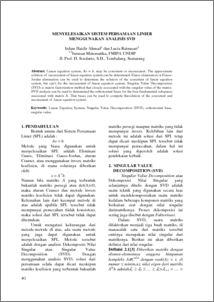# MENYELESAIKAN SISTEM PERSAMAAN LINIER MENGGUNAKAN ANALISIS SVD

Ahmad, Irdam Haidir and Ratnasari, Lucia (2010) MENYELESAIKAN SISTEM PERSAMAAN LINIER MENGGUNAKAN ANALISIS SVD. Jurnal Matematika, 13 (1). pp. 40-45. ISSN 1410-8518Preview
PDF
97Kb

## Abstract

Linear equation system, Ax = b, may be consistent or inconsistent. The approximate solution of inconsistent of linear equation system can be determined. Gauss elimination or Gauss-Jordan elimination can be used to determine the solution of the consistent of linear equation system, but can’t for the inconsistent of linear equation system. Singular Value Decomposition (SVD) is matrix factorization method that closely associated with the singular value of the matrix. SVD analysis can be used to determined the orthonormal bases for the four fundamental subspaces associated with matrix A. That bases can be used to compute thesolution of the consistent and inconsistent of linear equation system.

Item Type: Article Q Science > QA Mathematics Faculty of Science and Mathematics > Department of Mathematics 26873 Ms Niken P 19 Apr 2011 16:03 19 Apr 2011 16:03

Repository Staff Only: item control page